# 285 294 .pdf

### File information

Title: On the Exact Solution of Burgers-Huxley Equation Using the Homotopy Perturbation Method
Author: S. Salman Nourazar, Mohsen Soori, Akbar Nazari-Golshan

This PDF 1.7 document has been generated by Acrobat PDFMaker 11 Word ç‰ˆ / Adobe PDF Library 11.0, and has been sent on pdf-archive.com on 03/05/2017 at 06:30, from IP address 151.244.x.x. The current document download page has been viewed 979 times.
File size: 329.93 KB (10 pages).
Privacy: public file

### Document preview

Journal of Applied Mathematics and Physics, 2015, 3, 285-294
Published Online March 2015 in SciRes. http://www.scirp.org/journal/jamp
http://dx.doi.org/10.4236/jamp.2015.33042

On the Exact Solution of Burgers-Huxley
Equation Using the Homotopy Perturbation
Method
S. Salman Nourazar1, Mohsen Soori1*, Akbar Nazari-Golshan2
1

Department of Mechanical Engineering, Amirkabir University of Technology (Tehran Polytechnic),
Tehran, Iran
2
Department of Physics, Amirkabir University of Technology (Tehran Polytechnic), Tehran, Iran
*
*
Email: mohsen.soori@gmail.com, m.soori@aut.ac.ir
Received 3 March 2015; accepted 18 March 2015; published 23 March 2015

Abstract
The Homotopy Perturbation Method (HPM) is used to solve the Burgers-Huxley non-linear differential equations. Three case study problems of Burgers-Huxley are solved using the HPM and the
exact solutions are obtained. The rapid convergence towards the exact solutions of HPM is numerically shown. Results show that the HPM is efficient method with acceptable accuracy to solve
the Burgers-Huxley equation. Also, the results prove that the method is an efficient and powerful
algorithm to construct the exact solution of non-linear differential equations.

Keywords
Burgers-Huxley Equation, Homotopy Perturbation Method, Nonlinear Differential Equations

1. Introduction
Most of the nonlinear differential equations do not have an analytical solution. Recently, semi-analytical solutions of real-life mathematical modeling are considered as a key tool to solve nonlinear differential equations.
The idea of the Homotopy Perturbation Method (HPM) which is a semi-analytical method was first pioneered
by He . Later, the method is applied by He  to solve the non-linear non-homogeneous partial differential
equations. Nourazar et al.  used the homotopy perturbation method to find exact solution of Newell-WhiteheadSegel equation. Krisnangkura et al.  obtained exact traveling wave solutions of the generalized Burgers*

Corresponding author.

How to cite this paper: Nourazar, S.S., Soori, M. and Nazari-Golshan, A. (2015) On the Exact Solution of Burgers-Huxley
Equation Using the Homotopy Perturbation Method. Journal of Applied Mathematics and Physics, 3, 285-294.
http://dx.doi.org/10.4236/jamp.2015.33042

S. S. Nourazar et al.

Huxley equation by using hyperbolic tangent method. New exact solutions of the generalized Burgers-Huxley
equation are also obtained by Gao and Zhao . Hashim et al.  have solved the generalized Burgers-Huxley
equation by using adomian decomposition method. Wang et al.  studied the solitary wave solutions of the
generalized Burgers-Huxley equation. Also, Darvishi et al.  have used spectral collocation method and Darvishi’s preconditionings to solve the generalized Burgers-Huxley equation.
The generalized Burgers-Huxley equation is investigated by Satsuma  as:

∂u ∂ 2 u
∂u
=
− αun
+ β u 1 − u n u n − γ , 0 ≤ x ≤ 1, t ≥ 0
2
∂t ∂x
∂x

(

)(

)

(1.1)

where α , β ≥ 0 are real constants and n is a positive integer and γ ∈ [ 0,1] . Equation (1.1) models the interaction between reaction mechanisms, convection effects and diffusion transports .
When α = 0 and n = 1 , Equation (1.1) is reduced to the Huxley equation which describes nerve pulse propagationin nerve fibers and wall motion in liquid crystals . When β = 0 and n = 1 , Equation (1.1) is reduced to the Burgers equation describing the far field of wave propagation in nonlinear dissipative systems .
When n = 1 , α ≠ 0 and β ≠ 0 , Equation (1.1) is turned into the Burgers-Huxley equation. The Burgers-Huxley equation is used to model the interaction between reaction mechanisms, convection effects and diffusion
transport, nerve pulse propagation in nerve fibers as well as wall motion in liquid crystals.
In the present research work, the Homotopy Perturbation Method (HPM) is applied to obtain the closed form
solution of the non-linear Burgers-Huxley equation. Three case study problems of non-linear Burgers-Huxley
equations are solved by using the HPM. The trend of the rapid convergence towards the exact solution is shown
when compared to the exact solution.
The idea of homotopy perturbation method is presented in Section 2. Application of the homotopy perturbation method to the exact solution of Burgers-Huxley equation is presented in Section 3.

2. The Idea of Homotopy Perturbation Method
The Homotopy Perturbation Method (HPM) is originally initiated by He . This is a combination of the classical perturbation technique and homotopy technique. The basic idea of the HPM for solving nonlinear differential equations is as follow; consider the following differential equation:

E (u ) = 0 ,

(2.1)

where E is any differential operator. We construct a homotopy as follow:

H ( u, p ) =
(1 − p ) F ( u ) + p ( E ( u ) − F ( u ) ) .

(2.2)

where F ( u ) is a functional operator with the known solution v0 . It is clear that when p is equal to zero
u,1) E=
u , 0 ) F=
then H (=
( u ) 0 , and when p is equal to 1, then H (=
( u ) 0 . It is worth noting that as the
embedding parameter p increases monotonically from zero to unity the zero order solution v0 continuously
deforms into the original problem E ( u ) = 0 . The embedding parameter, p ∈ [ 0,1] , is considered as an expanding parameter . In the homotopy perturbation method the embedding parameter p is used to get series expansion for solution as:
u =∑ i = 0 p i vi =v0 + pv1 + p 2 v2 + p 3 v3 + 

(2.3)

when p → 1 , then Equation (2.2) becomes the approximate solution to Equation (2.1) as:

u = v0 + v1 + v2 + v3 +

(2.4)

The series Equation (2.4) is a convergent series and the rate of convergence depends on the nature of Equation
(2.1)  . It is also assumed that Equation (2.2) has a unique solution and by comparing the like powers of
p the solution of various orders is obtained. These solutions are obtained using the Maple package.

3. The Burgers-Huxley Equation
To illustrate the capability and reliability of the method, three cases of nonlinear diffusion equations are presented.

286

S. S. Nourazar et al.

α 0,=
γ 1,=
β 1 , so, the equn 1,=
Case І: in this case we will examine the Burgers-Huxley equation for =
ation is written as:
∂u ∂ 2 u
=
+ u (1 − u )( u − 1)
∂t ∂x 2

(3.1)

Subject to initial condition:
e

u ( x, 0 ) =
e

2
x
4

2
x
4

+e

(3.2)

2
x
4

We construct a homotopy for Equation (3.1) in the following form:

 ∂υ ∂u 
H (υ , p ) =(1 − p )  − 0  +
∂t 
 ∂t

 ∂υ ∂ 2υ

p  − 2 − υ (1 − υ )(υ − 1) 
t

x

(3.3)

The solution of Equation (3.1) can be written as a power series in p as:

υ =υ0 + pυ1 + p 2υ2 +

(3.4)

Substituting Equation (3.4) and Equation (3.2) into Equation (3.3) and equating the terms with identical powers
of p:
∂υ
p : 0
∂t
0

∂u0
,
=
υ 0 ( x, 0 )
∂t

∂υ ∂u
p1 : 1 + 0 =
∂t
∂t

∂ 2υ1
υ
p 2 : 2=
∂t
∂x 2
∂υ
∂ 2υ2
p 3 : 3=
∂t
∂x 2

e
e

∂ 2υ0
+ υ0 (1 − υ0 )(υ0 − 1) ,
∂x 2

υ1 ( x, 0 ) = 0,

+ 4υ0υ1 − υ1 − 3υ1υ02 ,

υ 2 ( x, 0 =
) 0,

2
x
4

2
x
4

+e

2
x
4

,

(3.5)

+ 4υ0υ2 − υ2 − 3υ2υ02 + 2υ12 − 3υ0υ12 , υ3 ( x, 0 =
) 0.

Using the Maple package to solve recursive sequences, Equation (3.5), we obtain the followings:
e

υ 0 ( x, t ) =
e

2
x
4

2
x
4

+e

2
x
4

, υ1 ( x, t ) = −

1
2
e

1
2
x
4

+e

2
x
4

2

t,

2

(3.6)

2

 2x − 2x 
 2x 
 − 2x 
e 4 −e 4 
e 4  − 4 +e 4 

1 
1 
 t 2 , υ x, t =

 t 3.
− 
υ 2 ( x, t ) =
) − 
3(
3
4
2
2
8  2x − 2x 
48

x
x
e 4 + e 4 
e 4 + e 4 

By setting p = 1 in Equation (3.4) the solution of Equation (3.1) can be obtained as υ = υ0 + υ1 + υ2 + υ3 +
Therefore the solution of Equation (3.1) is written as:

e

υ ( x, t ) =
2
e

4

x

2
x
4

+e

2

x
4

1
2
e

1
2
x
4

+e

2
x
4

2

 2x − 2x 

e 4 − e 4 
e

1 
1 

2
t−
t −
3
2 
8  2x
48

x
e 4 + e 4 

287

2
x
4

e

2

 −
 − 4 + e

2
x
4

+e

2
x
4

2
x
4

4

2

 t3 +

(3.7)

S. S. Nourazar et al.

t
2

x−

1 1 e 4 4 −e
The Taylor series expansion for  +
t
2
 2 2 e 4 x − 4 + e−

2
t
x−
4
4

1 1e
−e
+
2
t
2 2
x−

e 4 4 +e

2
t
x+
4
4
2
t
x+
4
4

e
=
2
e

4

x

2
x
4

+e

2
x

4

t
2
x+
4
4
t
2
x+
4
4

1
2
e

2

 is written as:

1
2
x
4

+e

2
x
4

2

2 
 2x

x
e 4 − e 4 

1
 t2
t− 
3
2 
8  2x
x

4
4
+e
e

(3.8)

2

 2x 
 − 2x 
e 4  − 4 +e 4 

1 

 t 3 + .
− 
4
2
2
48

x

x 
e 4 + e 4 

Combining Equation (3.8) with Equation (3.7), we get as follow:
2

x−

t

1 1 e 4 4 −e
υ ( x, t ) =
+
2 2 2 x− t

e 4 4 +e

2
t
x+
4
4
2
t
x+
4
4

 1 
1 1
t 
=
+ tanh 
x−

2 2
2 
2 2

(3.9)

This is the exact solution of the problem, Equation (3.1). Table 1 shows the trend of rapid convergence of the
results of S1 ( x, t ) = v0 ( x, t ) to S6 ( x, t ) = ∑ i = 0 vi ( x, t ) using the HPM. The rapid convergence of the solution
5

toward the exact solution, the maximum relative error of less than 0.0000058% is achieved as shown in Table 1.
Case ІІ: In Equation (1.1) for α =
−1, n =
1, γ =
1, β =
1 , the Burgers-Huxley equation is written as:

∂u ∂ 2 u
∂u
=
+ u + u (1 − u )( u − 1)
∂t ∂x 2
∂x

(3.10)

Table 1. The percentage of relative errors of the results of S1 ( x, t ) = v0 ( x, t ) to S6 ( x, t ) = ∑ i=0 vi ( x, t ) of the HPM solu5

tion of Equation (3.1).
Percentage of relative error (%RE)

t = 0.1

t = 0.3

t = 0.4

x =1

x=2

x=3

S1 ( x, t )

0.01693168743

0.01002710463

0.005488150424

S3 ( x, t )

0.000002337346256

2.025644856e−7

9.527700575e−7

S5 ( x, t )

2.153484215e−10

3.464089104e−10

3.155246333e−10

S 6 ( x, t )

9.744801271e−12

3.920421861e−11

9.937961084e−11

S1 ( x, t )

0.05344388963

0.03164997413

0.01732302882

S3 ( x, t )

0.00006806597676

0.000003967422423

0.00002580410708

S5 ( x, t )

6.184344787e−8

9.467317545e−8

5.516785201e−8

S 6 ( x, t )

1.008793018e−8

1.166422821e−9

2.026398250e−9

S1 ( x, t )

0.07311570399

0.04329980772

0.02369935005

S3 ( x, t )

0.0001675410515

0.000007498069748

0.00006123279256

S5 ( x, t )

2.799248652e−7

4.009848425e−7

2.364525175e−7

S 6 ( x, t )

5.775483086e−8

6.758498122e−9

1.111128458e−8

288

S. S. Nourazar et al.

Subject to initial condition:
e

u ( x, 0 ) =

x
4

x

e4 + e

(3.11)

x
4

To solve Equation (3.10) we construct a homotopy in the following form:

 ∂υ ∂u 
H (υ , p ) =(1 − p )  − 0  +
∂t 
 ∂t

 ∂υ ∂ 2υ

∂υ
p  − 2 −υ
− υ (1 − υ )(υ − 1) 
∂x
 ∂t ∂x

(3.12)

The solution of Equation (3.10) can be written as a power series in p as:

υ =υ0 + pυ1 + p 2υ2 +

(3.13)

Substituting Equation (3.13) and Equation (3.11) in to Equation (3.12) and equating the term with identical

∂υ
p : 0
∂t
0

∂u0
=
υ 0 ( x, 0 )
,
∂t

∂υ ∂u
p1 : 1 + 0 =
∂t
∂t
∂υ
∂ 2υ
p 2 : 2 = 21
∂t
∂x
∂υ
∂ 2υ
p 3 : 3 = 22
∂t
∂x

e

x
4

x
4

x
4

,

e +e
∂ 2υ0
∂υ0
+ υ0
+ υ0 (1 − υ0 )(υ0 − 1) ,
υ1 ( x, 0 ) = 0,
(3.14)
∂x
∂x 2
∂υ
∂υ
+ υ0 1 + υ1 0 + 4υ0υ1 − υ1 − 3υ1υ02 ,
υ2 ( x, 0 ) =0,
∂x
∂x
∂υ
∂υ
∂υ
+ υ0 2 + υ2 0 + υ1 1 + 4υ0υ2 − υ2 − 3υ2υ02 + 2υ12 − 3υ0υ12 , υ3 ( x, 0 ) =
0.
∂x
∂x
∂x

Using the Maple package to solve recursive sequences, Equation (3.14), we obtain the followings:

e

x
4

3
1
t,
x 2
4 x
− 
e +e
 e 4 + e 4 

  x 2  − x 2

x
− 
 4x
4
4

4
+

e
e
4

 e − e 
  

9 
9    

 t 3.
2
υ 2 ( x, t ) =
t , υ3 ( x, t ) = −
3
4
x
x
x
32  x
128
− 
− 
 4
 e 4 + e 4 
 e + e 4 

υ 0 ( x, t ) =

x
4

x
4

, υ1 ( x, t ) = −

(3.15)

By setting p = 1 in Equation (3.13), the solution of Equation (3.10) can be obtained as υ = υ0 + υ1 + υ2 + υ3 +
Therefore the solution of Equation (3.10) is written as:

x

e 4
υ ( x, t ) =
x
e +e
4

x
4

3
1
x 2
4 x
− 
4
4
 e + e 

  x 2  − x 2

x
− 
 4x
4
4

4
e
e
4
+

 e − e 
  

9 
9    

2
t+
t −
t3 +
x 3
x
x 4
32  x
128
− 
− 
 4
 e 4 + e 4 
 e + e 4 

x 3t
x 3t
+
− −

1 1 e4 8 − e 4 8

The Taylor series expansion for  −
x 3t
x 3t
 2 2 e 4 + 8 + e− 4 − 8

289

 is written as:

(3.16)

S. S. Nourazar et al.
x 3t
+
4 8

x 3t
− −
4 8

x

4

1 1e
−e
e

=
x 3t
x 3t
x
x
− −

2 2 4+ 8
e
+ e 4 8 e4 + e 4

  x 2  − x 2

x
− 
 4x

4
 e 4  +  e 4  − 4 
 e − e 

3
1
9 
 t2 − 9   
 t3 +

t
+
x 2
x 3
x 4
4 x
32  x
128
− 
− 
− 
 4x
4
4
4
4
4
e
+
e
e
+
e
e
+
e







(3.17)

By substituting Equation (3.17) into Equation (3.16), Equation (3.16) can be reduced to:
x 3t
+

x 3t
− −

1 1 e4 8 − e 4 8
1 1
3t  
1 

=
− tanh   x +  
υ ( x, t ) =
x 3t
x 3t
− −
2 2 4+ 8
2 2
2 
4 
+e 4 8
e

(3.18)

This is the exact solution of the problem, Equation (3.10). Table 2 shows the trend of rapid convergence of
5
the results of S1 ( x, t ) = v0 ( x, t ) to S6 ( x, t ) = ∑ i = 0 vi ( x, t ) using the HPM solution toward the exact solution.
The maximum relative error of less than 0.00014% is achieved in comparison to the exact solution as shown
in Table 2.
−2, n =
1, γ =
3, β =
1 , the Burgers-Huxley equation becomes:
Case ІІІ: In Equation (1.1) for α =

∂u ∂ 2 u
∂u
=
+ 2u + u (1 − u )( u − 3)
2
∂t ∂x
∂x

(3.19)

Subject to initial condition:
−3

u ( x, 0 ) =

3

e

(

3e

)x

(

3 −1
4

)x

3 −1
4

+e

(

3

(3.20)

)x

3 −1
4

Table 2. The percentage of relative errors of the results of S1 ( x, t ) = v0 ( x, t ) to S6 ( x, t ) = ∑ i=0 vi ( x, t ) of the HPM solu5

tion of Equation (3.10).
Percentage of relative error (%RE)

t = 0.1

t = 0.3

t = 0.4

x =1

x=2

x=3

S1 ( x, t )

0.0484797171

0.056937877

0.063676094

S3 ( x, t )

0.0000184239461

0.000009125432

0.000006989146

S5 ( x, t )

7.8094040e−9

3.9049212e−9

1.37134980e−8

S 6 ( x, t )

3.61301281e−10

6.1915442e−11

1.3095951e−10

S1 ( x, t )

0.1570606291

0.184462686

0.206292613

S3 ( x, t )

0.000524561340

0.00023856284

0.00024584133

S5 ( x, t )

0.00000177771365

0.00000129074423

0.00000380612758

S 6 ( x, t )

2.19608021e−7

2.10498615e−7

9.144252e−9

S1 ( x, t )

0.2177728801

0.255767283

0.2860356311

S3 ( x, t )

0.001277016710

0.00055367038

0.00065831599

S5 ( x, t )

0.0000075558572

0.0000060480463

0.0000170599007

S 6 ( x, t )

0.000001302473287

0.000001221861195

8.044206e−8

290

S. S. Nourazar et al.

We construct a homotopy for Equation (3.19) in the following form:

 ∂υ ∂u 
H (υ , p ) =(1 − p )  − 0  +
∂t 
 ∂t

 ∂υ ∂ 2υ

∂υ
p  − 2 − 2υ
− υ (1 − υ )(υ − 3) 
∂x
 ∂t ∂x

(3.21)

The solution of Equation (3.19) can be written as a power series in p as:

υ =υ0 + pυ1 + p 2υ2 +

(3.22)

Substituting Equation (3.22) and Equation (3.20) into Equation (3.21) and equating the terms with identical
powers of p:
−3

∂υ
p : 0
∂t
0

∂u0
υ 0 ( x, 0 )
=
,
∂t

3

(

e
∂υ
∂υ1 ∂u0 ∂ υ0
+
=
+ 2υ0 0 + υ0 (1 − υ0 )(υ0 − 3) ,
2
∂t
∂t
∂x
∂x
2
∂υ

υ
υ
υ
p 2 : 2 = 21 + 2υ0 1 + 2υ1 0 + 8υ0υ1 − 3υ1 − 3υ1υ02 ,
∂t
∂x
∂x
∂x
2
υ
υ

υ
υ

∂υ
p 3 : 3 = 22 + 2υ0 2 + 2υ2 0 + 2υ1 1 + 8υ0υ2 − 3υ2
∂t
∂x
∂x
∂x
∂x
2
2
2
− 3υ2υ0 + 4υ1 − 3υ0υ1 ,
2

p1 :

3e

)x

(

3 −1
4

)x

3 −1
4

+e

3

(

)x

3 −1
4

υ1 ( x, 0 ) = 0,

,

(3.23)

υ 2 ( x, 0 ) =
0,
υ3 ( x, 0 ) = 0.

Using the Maple package to solve recursive sequences, Equation (3.23), we obtain the followings:

(

−3

υ 0 ( x, t ) =

(

3

e

υ1 ( x, t ) = −

)x

)x

3 −1
4

3e
3 −1
4

+e

9
2  3(
e

(3
)x

(

3

4

3−4

3 −1
4

)x

3 −1

+e

(

3

,

)
)x 

3 −1
4

2

t,

 3( 3 −1) x − 3( 3 −1) x 
e 4
− e 4  43 − 24 3

27

υ 2 ( x, t ) = 
t2,
3
8
 3( 3 −1) x − 3( 3 −1) x 
e 4
+e 4 

(

27 
υ3 ( x, t ) = 
16  3(

 e



)

   3(
1

e
4 
3 −1)
3( 3 −1) 
 

x
x

4
+ e 4  
 
 

4

 − 3(
 − 4 +e

)x 

3 −1

2

2
)x  

3 −1
4

(

)

3
 389 − 225 3 t .

(3.24)

By setting p = 1 in Equation (3.22) the solution of Equation (3.19) can be obtained as υ = υ0 + υ1 + υ2 + υ3 +
Thus the solution of Equation (3.19) can be written as:

291

S. S. Nourazar et al.

 3( 3 −1) x − 3( 3 −1) x 
e 4
 43 − 24 3
−e 4
( )x

3
3
4
4
3e
9
27 

+
υ ( x, t ) =
t
t2
2
3
3( 3 −1)
3( 3 −1)
2
8
3( 3 −1) 
3( 3 −1) 
 3( 3 −1) x
 3( 3 −1) x
x
x

x
x

e 4
+e 4
e 4

e 4

+e 4
+e 4

−3

3 −1

27 
+ 
16   3(
 e



(

1

)x

3 −1
4

+e

3

(

)x 

3 −1
4

4

   3(

e
  

3

3 3e
The Taylor series expansion for  −
3
2 2e

3 3 − 3 9 3 −12
x+
t
4
4

3 3e

2 2 3
e

3 − 3 9 3 −12
x+
t
4
4

−e
+e

3 3 − 3 9 3 −12
x−
t
4
4

3 3 + 3 9 3 −12
x−
t
4
4

(

)

)x 

3 −1
4

 − 3(
 − 4 + e

2

3 − 3 9 3 −12
x+
t
4
4
3 − 3 9 3 −12
x+
t
4
4

−e
+e

2
)x  

3 −1
4

3 3 − 3 9 3 −12
x−
t
4
4

3 3 + 3 9 3 −12
x−
t
4
4

)

(

)

3
 389 − 225 3 t + .

 is written as:

 3( 3 −1) x − 3( 3 −1) x 
e 4
 43 − 24 3
−e 4

3 3−4
3e 4
9
27 

=

+
t
t2
2
3
3( 3 −1)
3( 3 −1)
2
8

3
3
1
3
3
1
3
3
1
3
3
1
(
)
)
(
(
)
)
(

x
x

x
x
x
x
+e 4
e 4
e 4

e 4

+e 4
+e 4

2

   3( 3 −1) 2
 − 3( 3 −1) x  
x
1
27 

 − 4 + e 4
  389 − 225 3 t 3 + .
e 4
+ 
4 

 
16   3( 3 −1)
3( 3 −1) 
  
x

x

 
4
4

+e
 e

 

 


(

−3

)x

3 −1

(

(3.25)

(

)

(

)

(3.26)

)

Comparing Equation (3.26) with Equation (3.25), thus Equation (3.25) can be reduced to:
3 3 −3

x+

9 3 −12

t

3 3 − 3 9 3 −12
x−
t
4
4

4
3 3e 4
−e

υ ( x, t ) =

3
3
3
9
3
12
3
2 2

x+
t
4
e 4
+e

3 + 3 9 3 −12
x−
t
4
4

3 3 − 3 
3 3
5 − 3 
=
− tanh 
t  
 x +
2 2
2
 4 
 

(3.27)

This is the exact solution of the problem, Equation (3.19). Table 3 shows the trend of rapid convergence of the
results of S1 ( x, t ) = v0 ( x, t ) to S6 ( x, t ) = ∑ i = 0 vi ( x, t ) using the HPM solution toward the exact solution. The
5

maximum relative error of less than 0.038% is achieved in comparison to the exact solution as shown in Table 3.
Deng  obtained some travelling solitary wave solutions of Equation (1.1) by applying the first-integral
method as follows:
1

γ γ
 nγ ( ρ  α ) 
  n
(α  ρ ) γ + (α ± ρ )( n + 1)
υ ( x, t ) =
x−
t + x0   
 ± tanh 

2 ( n + 1)
 2 2
  
 4 ( n + 1) 

292

(3.28)

S. S. Nourazar et al.

Table 3. The percentage of relative errors of the results of S1 ( x, t ) = v0 ( x, t ) to S6 ( x, t ) = ∑ i=0 vi ( x, t ) of the HPM solu5

tion of Equation (3.19).
Percentage of relative error (%RE)

t = 0.1

t = 0.3

t = 0.4

x =1

x=2

x=3

S1 ( x, t )

0.1473751972

0.1768549738

0.1894968996

S3 ( x, t )

0.00008115001396

0.0004703355304

0.0008489173163

S5 ( x, t )

5.853207295e−7

0.000001136972415

2.175815927e−7

S 6 ( x, t )

4.468762836e−8

5.878826669e−8

2.556253934e−8

S1 ( x, t )

0.5347070619

0.6416656548

0.6875331484

S3 ( x, t )

0.001445157793

0.01763206139

0.03053744832

S5 ( x, t )

0.0002129909216

0.0003484139717

0.00009299132649

S 6 ( x, t )

0.00003726778757

0.00005691935052

0.00002679413014

S1 ( x, t )

0.7871664200

0.9446250035

1.012148624

S3 ( x, t )

0.002172311991

0.04914756514

0.08362907281

S5 ( x, t )

0.001086228788

0.001651183957

0.0005006633300

S 6 ( x, t )

0.0002239213593

0.0003721014272

0.0001680489489

where

ρ = α 2 + 4 β (1 + n )

(3.29)

and x0 is arbitrary constant.
This is in full agreement of the closed form solutions of Equation (3.1), Equation (3.10) and Equation (3.19)
for differences value of parameters α , n, γ and β in the three cases. So, it can be concluded that the HPM is
a powerful and efficient technique to solve the non-linear Burgers-Huxley equation.

4. Conclusion
In the present research work, the exact solution of the Burgers-Huxley nonlinear diffusion equation is obtained
using the HPM. The validity and effectiveness of the HPM is shown by solving three non-homogenous non-linear
differential equations and the very rapid convergence to the exact solutions is also numerically demonstrated.
The trend of rapid and monotonic convergence of the solution toward the exact solution is clearly shown by obtaining the relative error in comparison to the exact solution. The rapid convergence towards the exact solutions
of HPM indicates that, using the HPM to solve the non-linear differential equations, a reasonable less amount of
computational work with acceptable accuracy may be sufficient. Moreover, it can be concluded that the HPM is
a very powerful and efficient technique which can construct the exact solution of nonlinear differential equations.

References


He, J.H. (1999) Homotopy Perturbation Technique. Computer Methods in Applied Mechanics and Engineering, 178,
257-262. http://dx.doi.org/10.1016/S0045-7825(99)00018-3



He, J.H. (2005) Application of Homotopy Perturbation Method to Nonlinear Wave Equations. Chaos Solitons &amp; Fractals, 26, 695-700. http://dx.doi.org/10.1016/j.chaos.2005.03.006



Nourazar, S.S., Soori, M. and Nazari-Golshan, A. (2011) On the Exact Solution of Newell-Whitehead-Segel Equation

293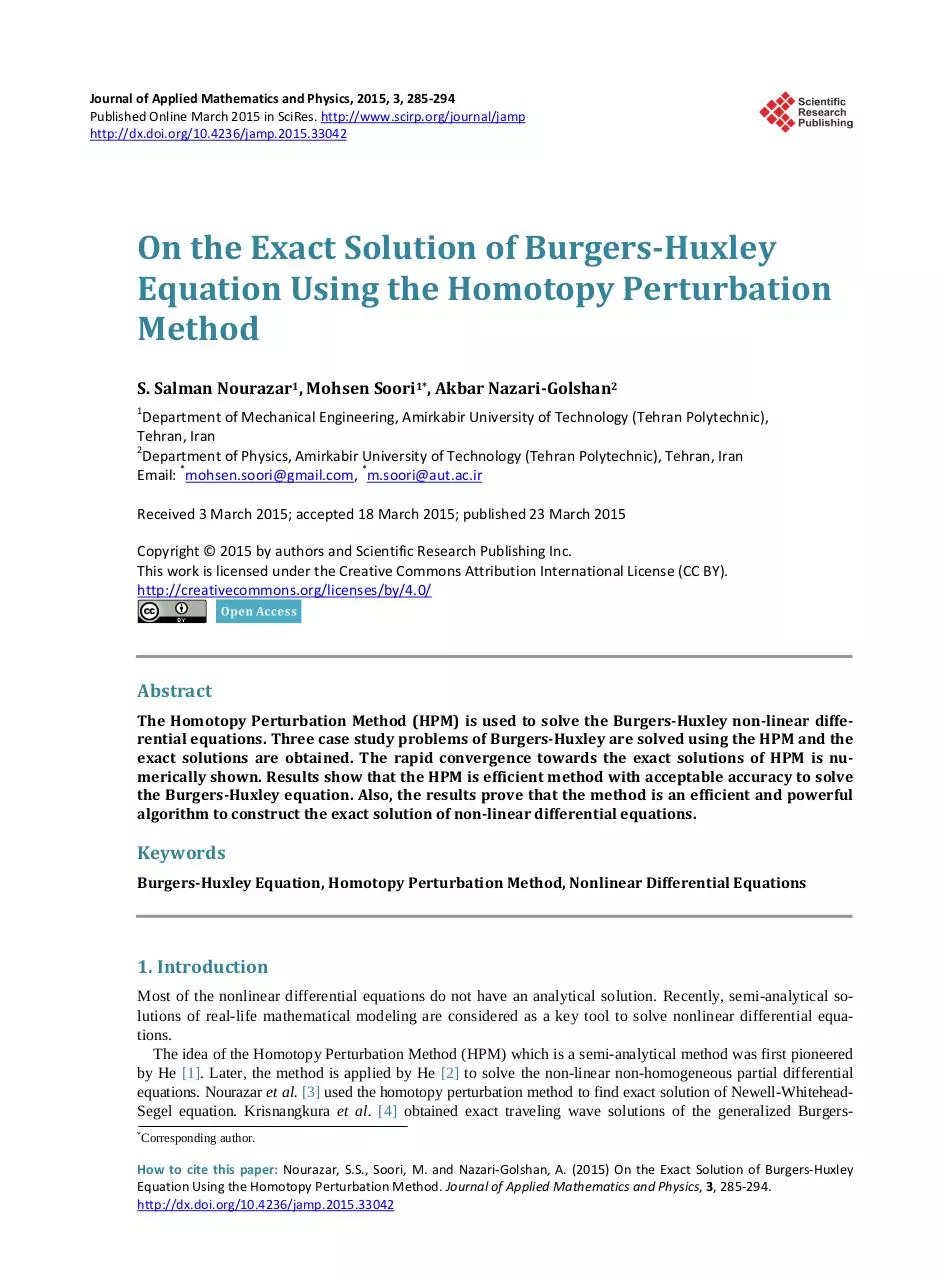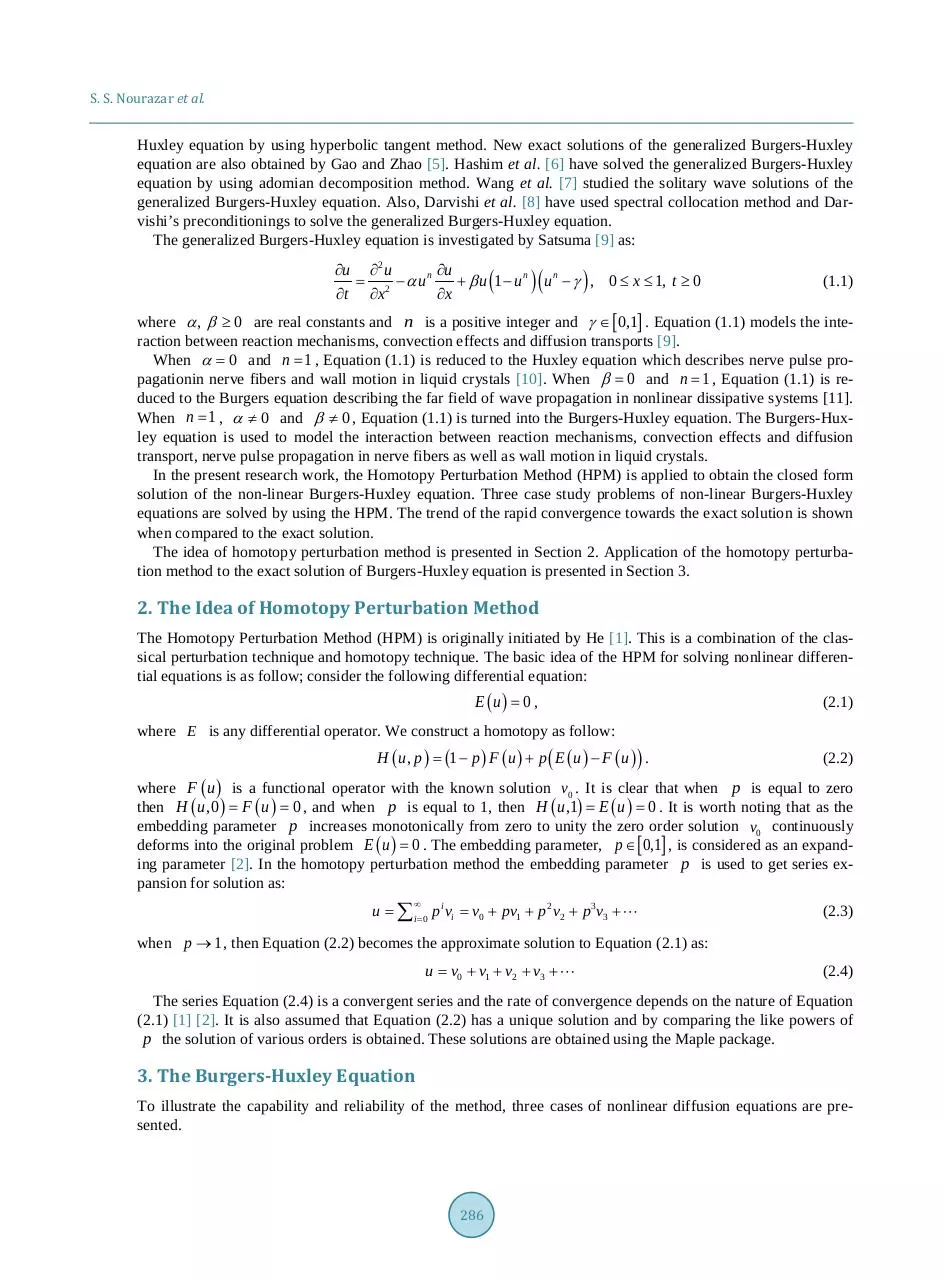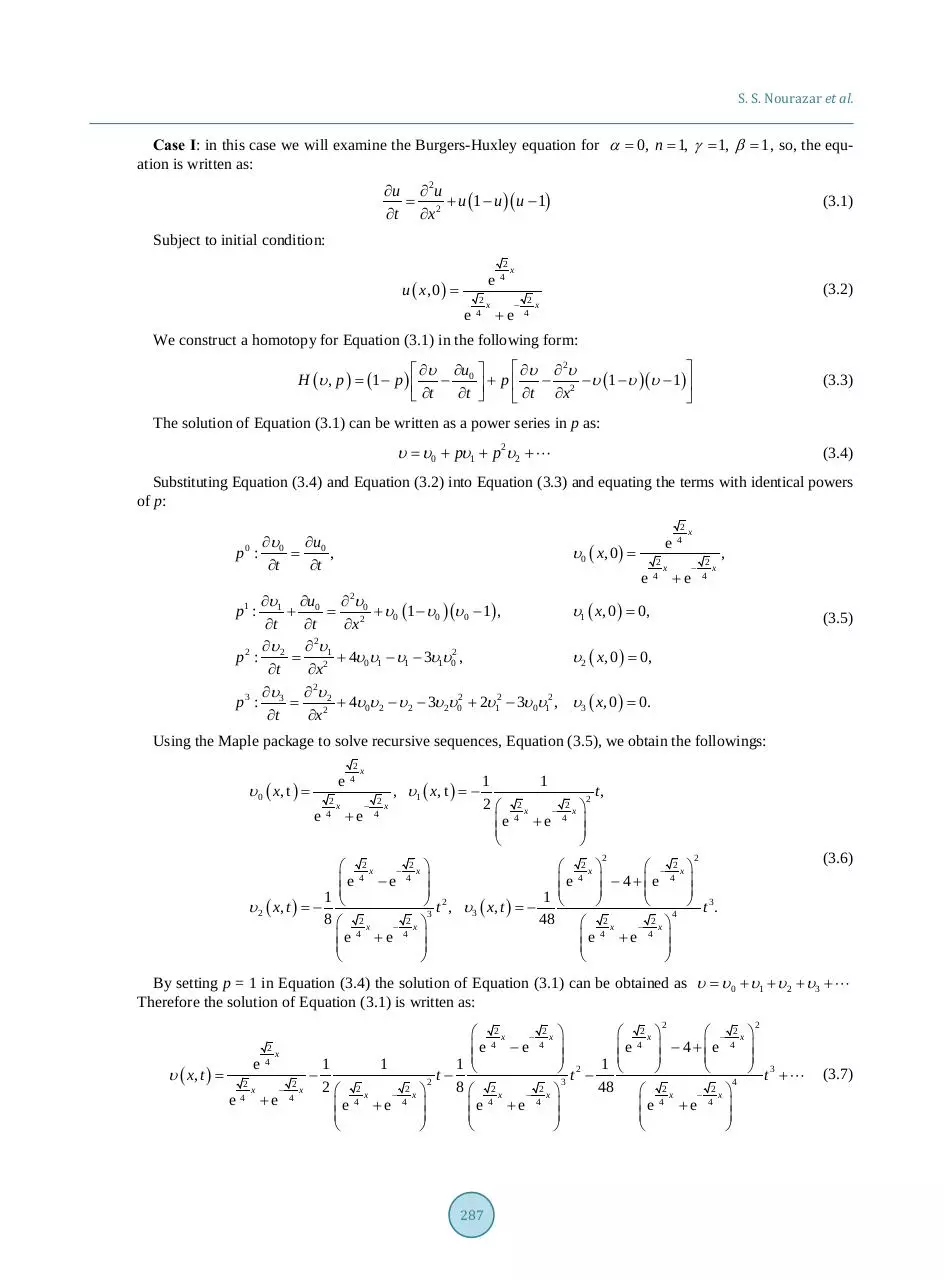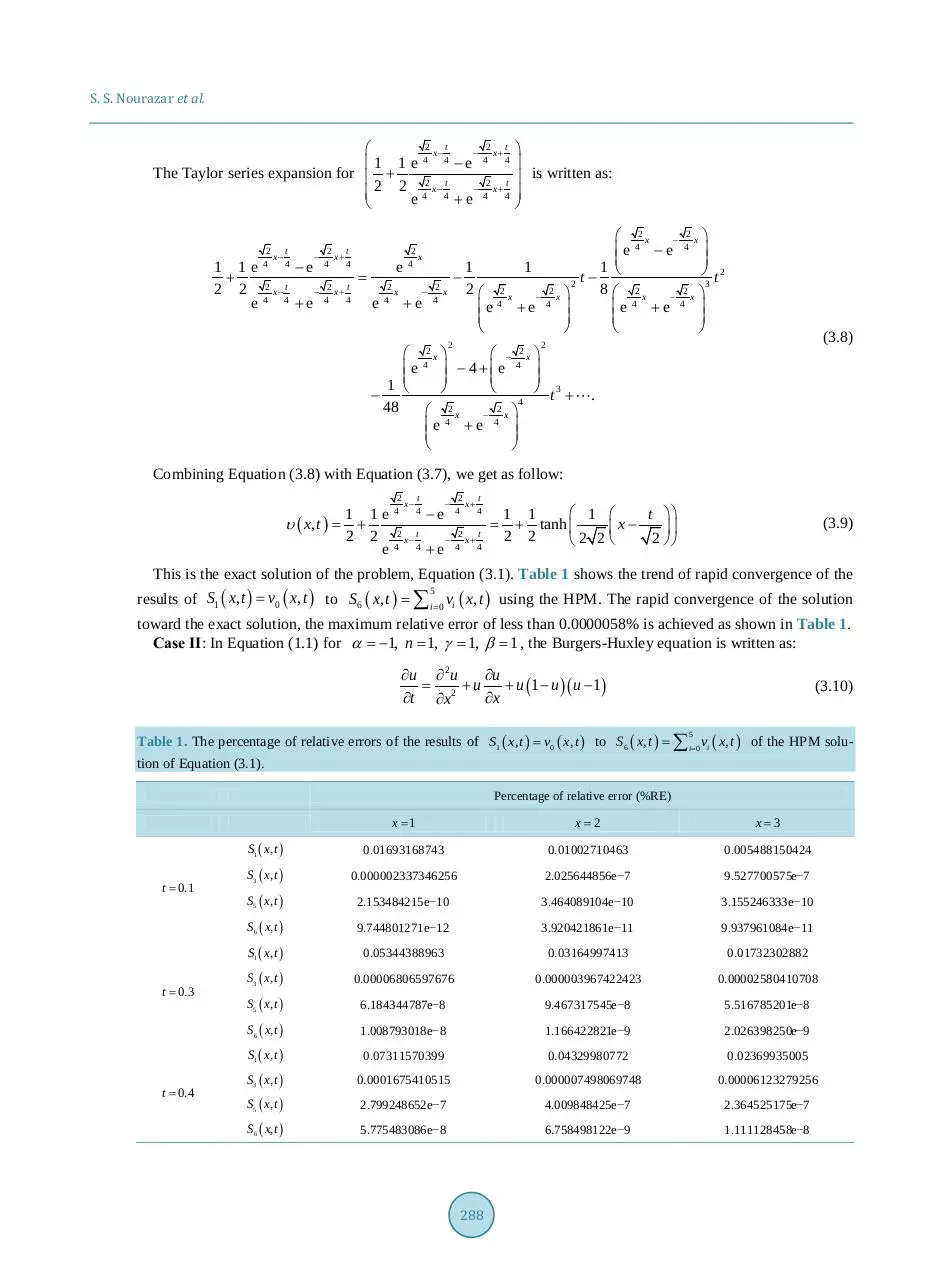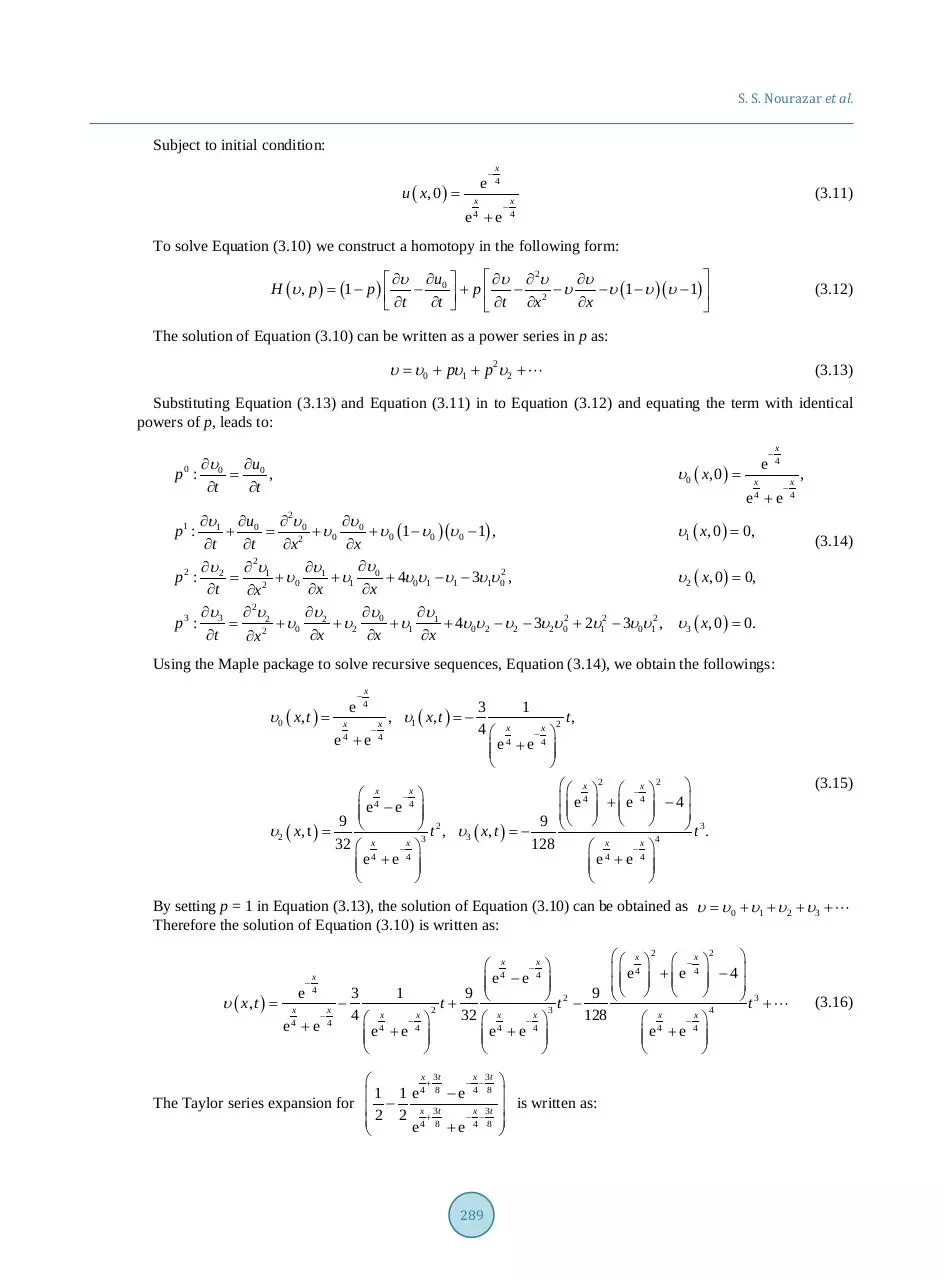285-294.pdf (PDF, 329.93 KB)

### Share on social networks

#### HTML Code

Copy the following HTML code to share your document on a Website or Blog